## 3.1.2 Visco-Elastic model using FEM

A visco-elastic oxide growth model has been developed at our institute using FEM as the simulation environment . The model is three-dimensional and does not rely on a simple expression for the interface motion, but rather relies on calculations of oxidant diffusion and oxide volume expansion in three dimensions to move the FEM mesh appropriately. A major limitation of FEM analysis is related to the mesh used for simulations. As either the time or space increments are decreased, or the mesh is made finer, the number of calculations necessary to simulate oxide growth can increase by a factor dependent on the model itself , . Some models also do not account for orientation dependence adequately or the error can be a non-linear function which can amplify significantly with further iterations .

The model presented in  is based on a few main equations. The first equation is meant to describe oxidant diffusion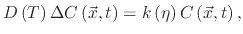(72)

where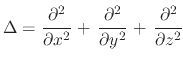is the Laplace operator,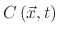is the oxidant concentration in the material, and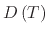is the temperature dependent low stress diffusion coefficient.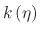is the strength of a spatial sink and not a simple reaction coefficient at a sharp interface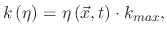(73)

where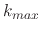is the maximal possible strength of the sink.

The next equation is meant to deal with the dynamics of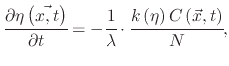(74)

where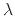is the volume expansion factor (=2.25) for the reaction from Si to SiO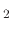, and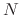is the number of oxidant molecules incorporated into a unit of SiOvolume.

The volume increase of the generating oxide occurs successively and not abruptly because of the diffuse interface concept. A volume increase of the oxidized material is calculated using theand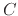values. After a given time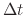, the normalized additional volume is given by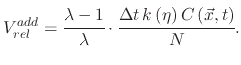(75)

An important aspect of (3.4) is that the sum of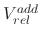over all time steps must not exceed 125%, which is the maximum volume expansion of the material during oxidation.

Since the principal axis components of the residual strain tensor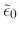are linearly proportional toin the form(76)

the normalized additional volume directly loads the mechanical problem. Using Hook's law, the stress tensor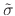is given by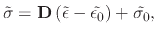(77)

where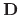is the so-called material matrix, constructed in Lame's form ,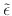is the strain tensor,is the residual strain tensor, and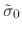is the residual stress tensor. The material matrix is rebuilt and (3.6) becomes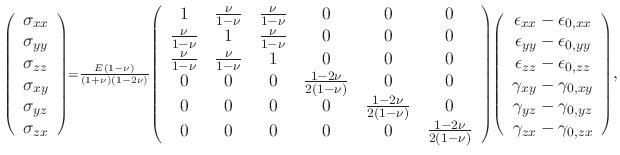(78)

where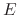is the Young's modulus,is the Poisson ratio, and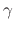are the shear strain components.

The effective shear modulus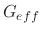can be added toto handle elastic and visco-elastic materials. For elastic materials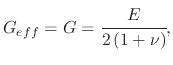(79)

while for visco-elastic materials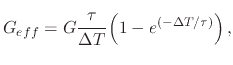(80)

where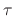is the Maxwellian relaxation time constant andis the effective shear modulus.

L. Filipovic: Topography Simulation of Novel Processing Techniques